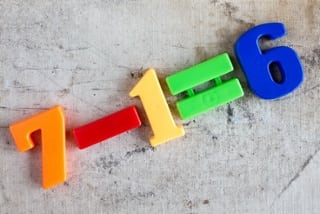## 4 Common Misconceptions Students Have About SubtractionGrade schoolers and teenagers alike have difficulty with subtraction. Sometimes these difficulties come from improper application of memorized rules, and sometimes they come from a lack of understanding of what subtraction actually means. So what makes this operation so tricky? Here are four common misconceptions that students have about subtraction:

1)      Only understanding subtraction as taking awayWhen first learning subtraction, students are taught that to subtract means to take away. This association allows learners to make a tangible connection. For example, the expression 9 – 3 can be modeled by the following scenario: if you have 9 pretzels, and you take away 3 pretzels, you are left with 6 pretzels. Therefore, 9 minus 3 is 6.

This definition works fine when subtracting a smaller positive number from a larger positive number, but it may cause trouble when students run into problems such as 4 – 5 or, even worse, 0 – 10. How can you subtract something from nothing? It can be difficult for some students to comprehend that even though they can’t physically “take away” another object, they can continue counting into negative values to arrive at a solution. This confusion can be avoided by coupling the idea of subtraction as taking away with the understanding that when you subtract two numbers, you are actually finding the difference between those two numbers.

2)      Confusing “negative” and “minus”

As described above, the concept of subtraction alone confuses many students. Once students begin studying algebra, they are encouraged to view subtraction as the inverse of addition. Now, the expression ab is equivalent to a + (-b), meaning a plus the opposite of b. Upon seeing “–” in a numeric expression, a new dilemma arises for some: does the symbol mean “minus,” “negative,” or “the opposite of”?

Consider the student who finds the sum of -4 and -6 incorrectly by stating -4 + -6 = 10. This could be interpreted as an improper application of the multiplication rule where “a negative and a negative equals a positive.” They fail to see that -4 + -6 is really just -4 – 6. Which leads us to another misconception…

3)      Subtracting negative numbersThe sign of a number indicates the direction in which to move along a number line. “+b” means move b units to the right, whereas “-b” means move b units to the left. Confusion entails when b itself is a negative value. Take 5 – (-3), for example. Some students start on the number line at 5 but only process the sign in front of the 3, meaning they move 3 in the negative direction, resulting in 2.

Misconceptions involving subtraction of negative numbers are perpetuated when students rely on previously learned procedures to evaluate these sorts of expressions. Middle school students are sometimes taught to address subtracting a negative value using “keep, change, change,” meaning keep the sign of the first number the same, change the minus sign to a plus sign, and change the negative number to a positive number. While this shortcut is probably introduced by educators with good intentions, it does not provide the student with the conceptual knowledge to understand why subtracting a negative number results in a positive value.

4)      Assuming subtraction is commutative

Since 5 + 4 and 4 + 5 both equal 9, it is understandable how students may assume that 10 – 25 and 25 – 10 are equivalent expressions. However, unlike its inverse operation, subtraction is NOT commutative. This misconception presents itself in secondary mathematics as well, when students start to write algebraic expressions from words. For example, it is all too common to see “5 less than 3x” written as 5 – 3x. Students correctly interpret “less than” to indicate subtraction, but do not realize that the 5 should be subtracted from 3x to produce the expression “5 less than 3x” and not the other way around.

These misconceptions can be overcome by ensuring that students have a solid mathematical understanding of subtraction in the early grades, so they do not have to rely on shortcuts or memorized algorithms later on.

## Who is A Pass?

A Pass Educational Group, LLC is an organization dedicated to the development of quality educational resources. We partner with publishers, K-12 schools, higher ed institutions, corporations, and other educational stakeholders to create custom quality content. Have questions?

Request Information
2019-08-09T13:57:17-05:00 August 19th, 2016|Course Writers, curriculum, mathematics, subtraction|0 Comments

# Subscribe to our blog!

Stay up-to-date on the latest Higher Ed, K-12 and Curriculum Design content.